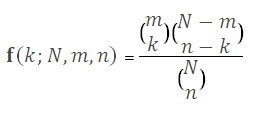# SQL Server HYPGEOM.DIST function

Updated: 9 August 2010

Use HYPGEOMDIST to calculate the hypergeometric distribution. HYPGEOMDIST calculates the probability of a given number of samples successes, given the sample size, population successes, and the population size. The formula for the hypergeometric distribution is:Where
k              is the number of successes in the sample
N             is the size of the sample
m            is the number of successes in the population
n             is the population size
Syntax
SELECT [wctStatistics].[wct].[HYPGEOMDIST] (
<@Sample_s, float,>
,<@Number_sample, float,>
,<@Population_s, float,>
,<@Number_population, float,>)
Arguments
@Sample_s
is the number of successes in the sample. @Sample_s is an expression of type float or of a type that can be implicitly converted to float
@Number_sample
is the size of the sample. @Number_sample  is an expression of type float or of a type that can be implicitly converted to float
@Population_s
is the number of successes in the population. @Population_s is an expression of type float or of a type that can be implicitly converted to float
@Number_population
is the population size. @Number_population is an expression of type float or of a type that can be implicitly converted to float
Return Types
float
Remarks
·         If @Sample_s < 0 or @Sample_s > MIN(@Number_sample, @Population_s), HYPGEOMDIST returns an error.
·         If @Sample_s < MAX(0, @Number_sample-@Number_population+@Population_s), HYPGEOMDIST returns an error.
·         If @Number_sample ≤ 0 or @Number_sample > @Number_population, HYPGEOMDIST returns an error.
·         If @Population_s ≤ 0 or @Population_s > @Number_populations, HYPGEOMDIST returns an error.
·         If @Number_population ≤ 0, HYPGEOMDIST returns an error.
Examples

select
wct.HYPGEOMDIST(20,900,45,2000)

This produces the following result
----------------------
0.120021812146482

(1 row(s) affected)

This value could also have been calculated using the FACTLN function.

select EXP((wct.FACTLN(45)-wct.FACTLN(25)-wct.FACTLN(45-25))+
(wct.FACTLN(1955)-wct.FACTLN(1075)-wct.FACTLN(1955-1075))-
(wct.FACTLN(2000)-wct.FACTLN(1100)-wct.FACTLN(2000-1100)))

This produces the following result
----------------------
0.120021812146482

(1 row(s) affected)

### SupportCopyright 2008-2023 Westclintech LLC         Privacy Policy        Terms of Service